Next: The electromagnetic energy tensor Up: Relativity and electromagnetism Previous: Relativistic particle dynamics

## The force on a moving charge

The electromagnetic 3-force acting on a charge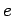moving with 3-velocity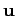is given by the well-known formula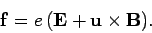(1556)

When written in component form this expression becomes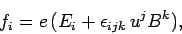(1557)

or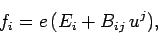(1558)

where use has been made of Eq. (1469).

Recall that the components of the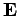and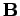fields can be written in terms of an antisymmetric electromagnetic field tensor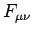via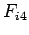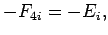(1559)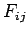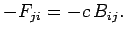(1560)

Equation (1558) can be written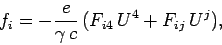(1561)

where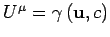is the particle's 4-velocity. It is easily demonstrated that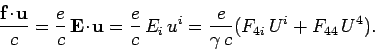(1562)

Thus, the 4-force acting on the particle,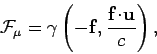(1563)

can be written in the form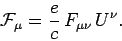(1564)

The skew symmetry of the electromagnetic field tensor ensures that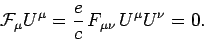(1565)

This is an important result, since it ensures that electromagnetic fields do not change the rest mass of charged particles. In order to appreciate this, let us assume that the rest mass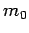is not a constant. Since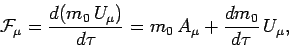(1566)

we can use the standard results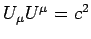and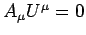to give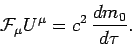(1567)

Thus, if rest mass is to remain an invariant, it is imperative that all laws of physics predict 4-forces acting on particles which are orthogonal to the particles' 4-velocities. The laws of electromagnetism pass this test.Next: The electromagnetic energy tensor Up: Relativity and electromagnetism Previous: Relativistic particle dynamics
Richard Fitzpatrick 2006-02-02